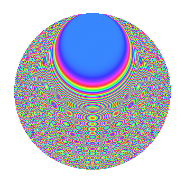# Properties

 Label 2100.2.ctLevel 2100 Weight 2 Character orbit ct Rep. character $$\chi_{2100}(113,\cdot)$$ Character field $$\Q(\zeta_{20})$$ Dimension 480 Sturm bound 960

# Related objects

## Defining parameters

 Level: $$N$$ $$=$$ $$2100 = 2^{2} \cdot 3 \cdot 5^{2} \cdot 7$$ Weight: $$k$$ $$=$$ $$2$$ Character orbit: $$[\chi]$$ $$=$$ 2100.ct (of order $$20$$ and degree $$8$$) Character conductor: $$\operatorname{cond}(\chi)$$ $$=$$ $$75$$ Character field: $$\Q(\zeta_{20})$$ Sturm bound: $$960$$

## Dimensions

The following table gives the dimensions of various subspaces of $$M_{2}(2100, [\chi])$$.

Total New Old
Modular forms 3936 480 3456
Cusp forms 3744 480 3264
Eisenstein series 192 0 192

## Trace form

 $$480q + 4q^{3} + O(q^{10})$$ $$480q + 4q^{3} - 24q^{13} - 8q^{15} - 40q^{19} - 88q^{25} - 8q^{27} + 20q^{33} - 32q^{37} + 40q^{39} + 8q^{43} - 20q^{45} + 8q^{55} + 28q^{57} + 32q^{63} + 104q^{67} + 80q^{73} + 28q^{75} + 80q^{79} - 40q^{81} + 184q^{85} + 120q^{87} + 104q^{97} + O(q^{100})$$

## Decomposition of $$S_{2}^{\mathrm{new}}(2100, [\chi])$$ into newform subspaces

The newforms in this space have not yet been added to the LMFDB.

## Decomposition of $$S_{2}^{\mathrm{old}}(2100, [\chi])$$ into lower level spaces

$$S_{2}^{\mathrm{old}}(2100, [\chi]) \cong$$ $$S_{2}^{\mathrm{new}}(75, [\chi])$$$$^{\oplus 6}$$$$\oplus$$$$S_{2}^{\mathrm{new}}(150, [\chi])$$$$^{\oplus 4}$$$$\oplus$$$$S_{2}^{\mathrm{new}}(300, [\chi])$$$$^{\oplus 2}$$$$\oplus$$$$S_{2}^{\mathrm{new}}(525, [\chi])$$$$^{\oplus 3}$$$$\oplus$$$$S_{2}^{\mathrm{new}}(1050, [\chi])$$$$^{\oplus 2}$$

## Hecke characteristic polynomials

There are no characteristic polynomials of Hecke operators in the database